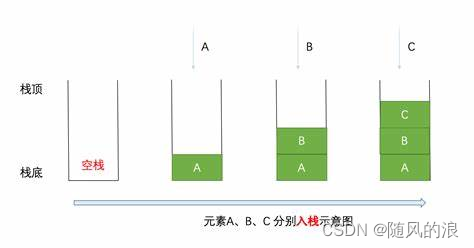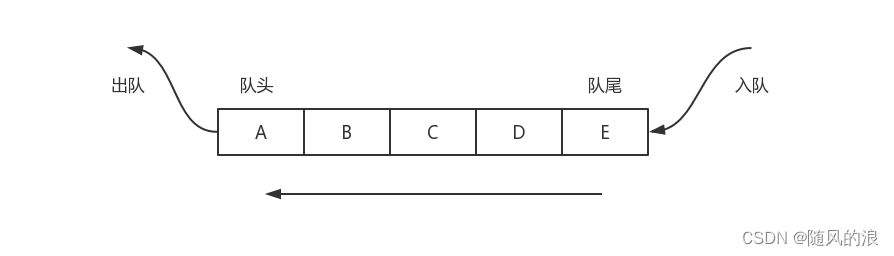# 关于栈和队列

### 目录

• 栈（Stack）
• 什么是栈
• 栈的使用
• 栈的模拟实现
• 队列（Queue）
• 什么是队列
• 队列的使用
• 队列的模拟实现
• 循环队列
• 双端队列 (Deque)

# 栈（Stack）

## 什么是栈## 栈的使用``````public static void main(String[] args) {
Stack<Integer> s = new Stack();  //尖括号里是我要存放的数据类型
s.push(1);
s.push(2);
s.push(3);
s.push(4);
System.out.println(s.size()); // 获取栈中有效元素个数---> 4
System.out.println(s.peek()); // 获取栈顶元素---> 4
s.pop(); // 4出栈，栈中剩余1 2 3，栈顶元素为3
System.out.println(s.pop()); // 3出栈，栈中剩余1 2 栈顶元素为2
if(s.empty()){
System.out.println("栈空");
}else{
System.out.println(s.size());
}
}
``````## 栈的模拟实现``````import java.util.Arrays;

public class MyStack {
int array[];     //数组用于存放数据
int size = 0;    //size用于记录当前元素个数

public MyStack() {
this(12);     //默认开辟大小为12的空间
}
public MyStack(int m) {
array = new int[m];
}
//入栈
public int push(int value) {
if(size == array.length) {
//扩容
int[] copy;
//复制array，并将其扩容一倍
copy = Arrays.copyOf(array,2* array.length);
array = copy;  //将array指向扩容后的数组
}
array[size] = value;
size++;
return value;
}
//出栈
public int pop() {
if(size == 0) {
throw new RuntimeException("栈中没有元素");
}
int n = array[size-1];
size--;
return n;
}
//获取栈顶元素
public int peek() {
if(size == 0) {
throw new RuntimeException("栈中没有元素");
}
return array[size-1];
}
//获取元素个数
public int getSize() {
return size;
}
//判断栈是否为空
public boolean isEmpty() {
return size == 0;
}
}

``````

# 队列（Queue）

## 什么是队列## 队列的使用``````	Queue<Integer> queue = new LinkedList<>();
````````````public static void main(String[] args) {
queue.offer(1);
queue.offer(2);
queue.offer(3);
queue.offer(4);
queue.offer(5); // 从队尾入队列
System.out.println(queue.size());  //获取元素个数
System.out.println(queue.peek()); // 获取队头元素
queue.poll();
System.out.println(queue.poll()); // 从队头出队列，并将删除的元素返回
if(queue.isEmpty()){
System.out.println("队列空");
}else{
System.out.println(queue.size());
}
}
``````## 队列的模拟实现

``````public class MyQueue {
//双向链表节点
public static class ListNode {
ListNode next;  //记录下一个节点
ListNode prev;  //记录上一个节点
int val;      //记录该节点存储的值
ListNode(int val){
this.val = val;
}
}
ListNode last; // 队尾
int size = 0;

//入队列
public void offer(int val) {
ListNode node = new ListNode(val);  //先实例化这个节点出来
if(last == null) {   //链表中未存放元素时
last = node;
}else {
last.next = node;
node.prev = last;
last = node;
}
size++;   //链表元素个数加一
}

//出队列
public int poll() {
if(isEmpty()) {  //判断队列是否为空
throw new RuntimeException("队列为空，无法出元素");
}
size--;
return m;
}

//判断队列是否为空
public boolean isEmpty() {
return size == 0;
}

//获取对头元素
public int peek() {
if(isEmpty()) {  //判断队列是否为空
throw new RuntimeException("队列为空，无法获取元素");
}
}

//获取元素个数
public int getSize() {
return size;
}
}
``````

## 循环队列

front 指向队列的第一个元素，即array[front]为队列的第一个元素，初始值为0；

rear 指向最后一个元素的后一个元素，初始值为0；1. 通过添加 size 属性记录（用size记录元素个数）
2. 牺牲一个位置来表示满``````class MyCircularQueue {
int front = 0;
int rear = 0;
int[] array;
public MyCircularQueue(int k) {  //设计一个可以存放k个数据的数组
array = new int[k+1];     //为什么要设计k+1个大小呢？
}                           //因为在队尾后面需要牺牲一个空间，方便判满

//向循环队列插入一个元素。如果成功插入则返回真。
public boolean enQueue(int value) {
if(isFull()) {
return false;
}
array[rear] = value;
rear = (rear + 1) % array.length;
return true;
}

//从循环队列中删除一个元素。如果成功删除则返回真。
public boolean deQueue() {
if(isEmpty()) {
return false;
}
front = (front + 1) % array.length;
return true;
}

//从队首获取元素。如果队列为空，返回 -1 。
public int Front() {
if(isEmpty()) {
return -1;
}
return array[front];
}

//获取队尾元素。如果队列为空，返回 -1 。
public int Rear() {
if(isEmpty()) {
return -1;
}
return array[(rear+array.length-1)%array.length];
}

//检查循环队列是否为空。
public boolean isEmpty() {
if(rear == front) {
return true;
}
return false;
}

//检查循环队列是否已满。
public boolean isFull() {
if((rear+1) % array.length == front) {
return true;
}
return false;
}
}
``````

## 双端队列 (Deque)

``````	Deque<Integer> stack = new ArrayDeque<>();//双端队列的线性实现